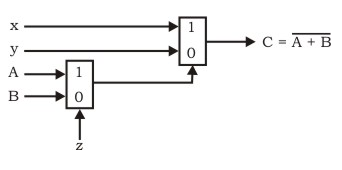Related Articles

# GATE | Gate IT 2005 | Question 48

• Last Updated : 19 Nov, 2018

The circuit shown below implements a 2-input NOR gate using two 2-4 MUX (control signal 1 selects the upper input). What are the values of signals x, y and z?(A) 1, 0, B
(B) 1, 0, A
(C) 0, 1, B
(D) 0, 1, A

Explanation:
In MUX1, equation is : g = Az + Bz’
In MUX2, equation is : f = xg + yg’ = x(Az + Bz’) + y (Az + Bz’)’

Function ‘f’ should be equal to (A + B)’ .

Using hit and try method, put x = 0, y = 1 and z = A.
f = 0(AA + BA’) + 1(AA + BA’)’
f = (A + A’B)’
f = (A + B)’

Thus, option (D) is correct.

Please comment below if you find anything wrong in the above post.

Quiz of this Question

Attention reader! Don’t stop learning now.  Practice GATE exam well before the actual exam with the subject-wise and overall quizzes available in GATE Test Series Course.

Learn all GATE CS concepts with Free Live Classes on our youtube channel.

My Personal Notes arrow_drop_up